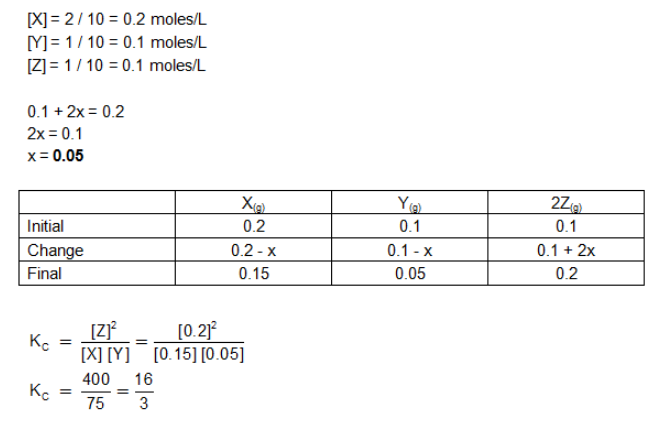# In the reaction X(g)+Y(g)⇔2Z(g), 2 mole of X,1 mole of Y and 1 in a 10-liter

In the reaction X(g)+Y(g)⇔2Z(g), 2 mole of X,1 mole of Y and 1 mole of Z are placed in a 10-liter vessel and allowed to reach equilibrium. If final concentration of Z is 0.2 M,Then Kc for the given reaction is :
a. 1.60
b. 80/3
c. 16/3
d. None of these